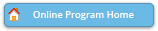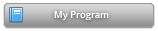#### Abstract Details

 Activity Number: 72 - Semiparametric Modeling Type: Contributed Date/Time: Sunday, July 28, 2019 : 4:00 PM to 5:50 PM Sponsor: Section on Nonparametric Statistics Abstract #302924 Title: Identification and Estimation of a Semiparametric Single Index Transformation Model Author(s): Yingqian Lin* and Yundong Tu Companies: Guanghua School of Management and Peking University, Guanghua School of Management and Center for Statistical Science Keywords: Nonparametric identification; Kernel estimation; Sieve method; Single index; Transformation models Abstract: In this paper, we consider a semiparametric single index model in which the dependent variable is subject to a nonparametric transformation. The model has the form G(Y)=g(X'\theta)+e, where X is a random vector of regressors, Y is the dependent variable and e is the random noise, the monotonic function G, the smooth function g and the vector \theta are all unknown. This model is quite general in the sense that it nests many popular regression models as special cases. We first propose identification strategies for the three unknown quantities, based on which estimators are then constructed. The density weighted average derivative estimator of \delta (proportional to \theta) is shown to be a V-statistic, which converges at sqrt{n}-rate and is asymptotically normal. The estimator of the transformation function G is a functional of the conditional distribution estimator of Y given X and is shown to be sqrt{n}-consistent and asymptotically normal. The estimator of g is shown to enjoy the standard nonparametric asymptotic properties. Simulation studies illustrate the nice finite sample performance of the proposed estimators, and a real data example demonstrates the practical merit.

Authors who are presenting talks have a * after their name.